## Construct and Run a Stateflow Chart

A Stateflow® chart is a graphical representation of a finite state machine consisting of states, transitions, and data. You can create a Stateflow chart to define how a MATLAB® algorithm or a Simulink® model reacts to external input signals, events, and time-based conditions. For more information, see Model Finite State Machines.

For instance, this Stateflow chart presents the logic underlying a half-wave rectifier. The chart contains two states labeled `On` and `Off`. In the `On` state, the chart output signal `y` is equal to the input `x`. In the `Off` state, the output signal is set to zero. When the input signal crosses some threshold `t0`, the chart transitions between these states. The actions in each state update the value of `y` at each time step of the simulation.This example shows how to create this Stateflow chart for simulation in Simulink and execution in MATLAB.

### Construct the Stateflow Chart

#### Open the Stateflow Editor

The Stateflow Editor is a graphical environment for designing state transition diagrams, flow charts, state transition tables, and truth tables. Before opening the Stateflow Editor, decide on the chart execution mode that best meets your needs.

• To model conditional, event-based, and time-based logic for periodic or continuous-time Simulink algorithms, use the `sfnew` function to create a Stateflow chart that you can simulate as a block in a Simulink model. At the MATLAB command prompt, enter:

`sfnew rectify % create chart for simulation in a Simulink model`

Simulink creates a model called `rectify` that contains an empty Stateflow Chart block. To open the Stateflow Editor, double-click the chart block.

• To design reusable state machine and timing logic for MATLAB applications, use the `edit` function to create a standalone Stateflow chart that you can execute as a MATLAB object. At the MATLAB command prompt, enter:

`edit rectify.sfx % create chart for execution as a MATLAB object`

If the file `rectify.sfx` does not exist, the Stateflow Editor creates an empty chart with the name `rectify`.

The main components of the Stateflow Editor are the chart canvas, the object palette, and the Symbols pane.

• The chart canvas is a drawing area where you create a chart by combining states, transitions, and other graphical elements.

• On the left side of the canvas, the object palette displays a set of tools for adding graphical elements to your chart.

• On the right side of the canvas, in the Symbols pane, you add new data, events, and messages to the chart and resolve any undefined or unused symbols.Tip

After you construct your Stateflow chart, you can copy its contents to another chart with a different execution mode. For example, you can construct a chart for execution in MATLAB and copy its contents into a chart for simulation in Simulink.

1. From the object palette, click the icon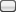and move the pointer to the chart canvas. A state with its default transition appears. To place the state, click a location on the canvas. At the text prompt, enter the state name `On` and the state action `y = x`.2. Add another state. Right-click and drag the `On` state. Blue graphical cues help you to align your states horizontally or vertically. The name of the new state changes to `Off`. Double-click the state and modify the state action to `y = 0`.3. Realign the two states and pause on the space between the two states. Blue transition cues indicate several ways in which you can connect the states. To add transitions, click the appropriate cue.

Alternatively, to draw a transition, click and drag from the edge of one state to the edge of the other state.4. Double-click each transition and type the appropriate transition condition `x<t0` or `x>=t0`. The conditions appear inside square brackets.5. Clean up the chart:

• To improve clarity, move each transition label to a convenient location above or below its corresponding transition.

• To align and resize the graphical elements of your chart, in the Format tab, click Auto Arrange or press Ctrl+Shift+A.

• To resize the chart to fit the canvas, press the space bar or click the icon.

#### Resolve Undefined Symbols

Before you can execute your chart, you must define each symbol that you use in the chart and specify its scope (for example, input data, output data, or local data). In the Symbols pane, undefined symbols are marked with a red error badge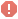. The Type column displays the suggested scope for each undefined symbol based on its usage in the chart.

1. Open the Symbols pane.

• If you are building a chart in a Simulink model, in the Modeling tab, under Design Data, select .

• If you are building a standalone chart for execution in MATLAB, in the State Chart tab, select Add Data > Symbols Pane.

2. In the Symbols pane, click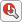.

• If you are building a chart in a Simulink model, the Stateflow Editor resolves the symbols `x` and `t0` as input dataand `y` as output data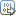.

• If you are building a standalone chart for execution in MATLAB, the Stateflow Editor resolves `t0`, `x`, and `y` as local data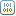.3. Because the threshold `t0` does not change during simulation, change its scope to constant data. In the Type column, click the data type icon next to `t0` and select`Constant Data`.

4. Set the value for the threshold `t0`. In the Value column, click the blank entry next to `t0` and enter a value of 0.

### Simulate the Chart as a Simulink Block

To simulate the chart inside a Simulink model, connect the chart block to other blocks in the model through input and output ports. If you want to execute the chart from the MATLAB Command Window, see Execute the Chart as a MATLAB Object.

1. To return to the Simulink Editor, on the explorer bar at the top of the canvas, click the name of the Simulink model:`rectify`. If the explorer bar is not visible, click the iconat the top of the object palette.

2. Add a source to the model:

• Double-click the Sine Wave block and set the Sample time to 0.2.

• Connect the output of the Sine Wave block to the input of the Stateflow chart.

• Label the signal as `x`.

3. Add a sink to the model:

• Connect the output of the Sine Wave block to the first input of the Scope block.

• Connect the output of the Stateflow chart to the second input of the Scope block.

• Label the signal as `y`.5. To simulate the model, click. During the simulation, the Stateflow Editor highlights active states and transitions through chart animation.

6. After you simulate the model, double-click the Scope block. The scope displays the graphs of the input and output signals to the charts.The simulation results show that the rectifier filters out negative input values.

### Execute the Chart as a MATLAB Object

To execute the chart in the MATLAB Command Window, create a chart object and call its `step` function. If you want to simulate the chart inside a Simulink model, see Simulate the Chart as a Simulink Block.

1. Create a chart object `r` by using the name of the `sfx` file that contains the chart definition as a function. Specify the initial value for the chart data `x` as a name-value pair.

`r = rectify(x=0);`
2. Initialize input and output data for chart execution. The vector `X` contains input values from a sine wave. The vector `Y` is an empty accumulator.

```T = 0:0.2:10; X = sin(T); Y = [];```
3. Execute the chart object by calling the `step` function multiple times. Pass individual values from the vector `X` as chart data `x`. Collect the resulting values of `y` in the vector `Y`. During the execution, the Stateflow Editor highlights active states and transitions through chart animation.

```for i = 1:51 step(r,x=X(i)); Y(i) = r.y; end```
4. Delete the chart object `r` from the MATLAB workspace.

`delete(r)`
5. Examine the results of the chart execution. For example, you can call the `stairs` function to create a stairstep graph that compares the values of `X` and `Y`.

```ax1 = subplot(2,1,1); stairs(ax1,T,X,color="#0072BD") title(ax1,"x") ax2 = subplot(2,1,2); stairs(ax2,T,Y,color="#D95319") title(ax2,"y")```The execution results show that the rectifier filters out negative input values.